1. x + 8 = -5 -13 -3 3 13 2. 7x - 1 = 6x - 4 -5 5 -3 -3/13 3. 7(x - 3) = 2 + 6(x - 5) -10 1 -14 -7 4. 9x - 5 = 8x 0 5 -5 5/17 5. 5x - 30 + 3x = 10 + 3x -20 4/3 6 4 -6 6.9 25 30 -9 7. 7x = -14 -7 2 -2 -21 8.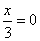3 -3 0 undefined 9. -6x = 48 54 42 -8 8 10. 8 - 3x = 17 -3 -25/3 3 6 11. 11x - 5 = 3x + 2 7/8 -3/8 15 1 12. 8x - 5 = 6x - 5 3 0 -2 undefined 13. 6(2x + 1) - 5x = 3x + 2 3/4 2 -1 1/2 14. x - 2 >5 x < 7 x > 7 x < 3 x > 3 15. 3 - x < 4 x >1 x < 1 x > -1 x < -1 16. 18 is 3 less than a number. What is the number? 15 -15 21 6 17. The sum of three consecutive numbers is 108. What is the largest number? 35 37 36 38 18. Chloe jogged 3 times as many miles as Jake, while Jossie jogged 2 miles less than Jake. If the three ran a total of 18 miles, how far did Jossie go? 4 2 12 3 19. The length of a rectangle is 2 less than 3 times the width. The perimeter is 60 inches. Find the width. 8 inches 22 inches 15.5 inches 20.7 inches 20. Twice a number decreased by 7 is 1. What is the number? -3 -4 4 6 21. 3 - (2x + 5) = 4 -3 -3/2 -11/6 2 22. 7 - 2x < 21 x < 7 x > 7 x < -7 x > -7 23. Solve for x if ax + by = c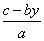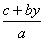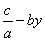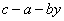24. Solve for x if y = mx + b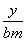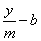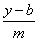25. Solve for t if I = prt Ipr I/(pr) I/(pt) I/(rt) 26. Solve for W if P= 2L+2W (P-2L)/2 (P-L)/2 (2L-P)/2 (P+2L/2